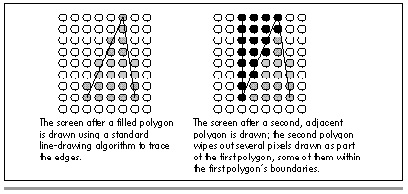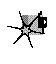How Do You Fit Polygons Together?

How, then, do you fit polygons together? Very carefully. First, the line-tracing algorithm must be adjusted so that it selects only those pixels that are truly inside the polygon. This basically requires shifting a standard line-drawing algorithm horizontally by one half-pixel toward the polygon’s interior. That leaves the issue of how to handle points that are exactly on the boundary, and points that lie at vertices, so that those points are drawn once and only once. To deal with that, we’re going to adopt the following rules:

Points located exactly on nonhorizontal edges are drawn only if the interior of the polygon is directly to the right (left edges are drawn, right edges aren’t).Figure 38.3

Points located exactly on horizontal edges are drawn only if the interior of the polygon is directly below them (horizontal top edges are drawn, horizontal bottom edges aren’t).
A vertex is drawn only if all lines ending at that point meet the above conditions (no right or bottom edges end at that point).

All edges of a polygon except those that are flat tops or flat bottoms will be considered either right edges or left edges, regardless of slope. The left edge is the one that starts with the leftmost line down from the top of the polygon.

These rules ensure that no pixel is drawn more than once when adjacent polygons are filled, and that if polygons cover the full 360-degree range around a pixel, then that pixel will be drawn once and only once—just what we need in order to be able to fit filled polygons together seamlessly.This sort of non-overlapping polygon filling isn’t ideal for all purposes. Polygons are skewed toward the top and left edges, which not only introduces drawing error relative to the ideal polygon but also means that a filled polygon won’t match the same polygon drawn unfilled. Narrow wedges and one-pixel-wide polygons will show up spottily. All in all, the choice of polygon-filling approach depends entirely on the ways in which the filled polygons must be used.

For our purposes, nonoverlapping polygons are the way to go, so let’s have at them.

Filling Non-Overlapping Convex Polygons

Without further ado, Listing 38.1 contains a function, FillConvexPolygon, that accepts a list of points that describe a convex polygon, with the last point assumed to connect to the first, and scans it into a list of lines to fill, then passes that list to the function DrawHorizontalLineList in Listing 38.2. Listing 38.3 is a sample program that calls FillConvexPolygon to draw polygons of various sorts, and Listing 38.4 is a header file included by the other listings. Here are the listings; we’ll pick up discussion on the other side.

LISTING 38.1 L38-1.C

/* Color-fills a convex polygon. All vertices are offset by (XOffset,
YOffset). “Convex” means that every horizontal line drawn through
the polygon at any point would cross exactly two active edges
(neither horizontal lines nor zero-length edges count as active
edges; both are acceptable anywhere in the polygon), and that the
right & left edges never cross. (It’s OK for them to touch, though,
so long as the right edge never crosses over to the left of the
left edge.) Nonconvex polygons won’t be drawn properly. Returns 1
for success, 0 if memory allocation failed. */

#include <stdio.h>
#include <math.h>
#ifdef __TURBOC__
#include <alloc.h>
#else    /* MSC */
#include <malloc.h>
#endif
#include “polygon.h”

/* Advances the index by one vertex forward through the vertex list,
wrapping at the end of the list */
#define INDEX_FORWARD(Index) \
Index = (Index + 1) % VertexList->Length;

/* Advances the index by one vertex backward through the vertex list,
wrapping at the start of the list */
#define INDEX_BACKWARD(Index) \
Index = (Index - 1 + VertexList->Length) % VertexList->Length;

/* Advances the index by one vertex either forward or backward through
the vertex list, wrapping at either end of the list */
#define INDEX_MOVE(Index,Direction)                                  \
if (Direction > 0)                                                \
Index = (Index + 1) % VertexList->Length;                      \
else                                                              \
Index = (Index - 1 + VertexList->Length) % VertexList->Length;

extern void DrawHorizontalLineList(struct HLineList *, int);
static void ScanEdge(int, int, int, int, int, int, struct HLine **);

int FillConvexPolygon(struct PointListHeader * VertexList, int Color,
int XOffset, int YOffset)
{
int i, MinIndexL, MaxIndex, MinIndexR, SkipFirst, Temp;
int MinPoint_Y, MaxPoint_Y, TopIsFlat, LeftEdgeDir;
int NextIndex, CurrentIndex, PreviousIndex;
int DeltaXN, DeltaYN, DeltaXP, DeltaYP;
struct HLineList WorkingHLineList;
struct HLine *EdgePointPtr;
struct Point *VertexPtr;

/* Point to the vertex list */
VertexPtr = VertexList->PointPtr;

/* Scan the list to find the top and bottom of the polygon */
if (VertexList->Length == 0)
return(1);  /* reject null polygons */
MaxPoint_Y = MinPoint_Y = VertexPtr[MinIndexL = MaxIndex = 0].Y;
for (i = 1; i < VertexList->Length; i++) {
if (VertexPtr[i].Y < MinPoint_Y)
MinPoint_Y = VertexPtr[MinIndexL = i].Y; /* new top */
else if (VertexPtr[i].Y > MaxPoint_Y)
MaxPoint_Y = VertexPtr[MaxIndex = i].Y; /* new bottom */
}
if (MinPoint_Y == MaxPoint_Y)
return(1);  /* polygon is 0-height; avoid infinite loop below */

/* Scan in ascending order to find the last top-edge point */
MinIndexR = MinIndexL;
while (VertexPtr[MinIndexR].Y == MinPoint_Y)
INDEX_FORWARD(MinIndexR);
INDEX_BACKWARD(MinIndexR); /* back up to last top-edge point */

/* Now scan in descending order to find the first top-edge point */
while (VertexPtr[MinIndexL].Y == MinPoint_Y)
INDEX_BACKWARD(MinIndexL);
INDEX_FORWARD(MinIndexL); /* back up to first top-edge point */

/* Figure out which direction through the vertex list from the top
vertex is the left edge and which is the right */
LeftEdgeDir = -1; /* assume left edge runs down thru vertex list */
if ((TopIsFlat = (VertexPtr[MinIndexL].X !=
VertexPtr[MinIndexR].X) ? 1 : 0) == 1) {
/* If the top is flat, just see which of the ends is leftmost */
if (VertexPtr[MinIndexL].X > VertexPtr[MinIndexR].X) {
LeftEdgeDir = 1;  /* left edge runs up through vertex list */
Temp = MinIndexL;       /* swap the indices so MinIndexL   */
MinIndexL = MinIndexR;  /* points to the start of the left */
MinIndexR = Temp;       /* edge, similarly for MinIndexR   */
}
} else {
/* Point to the downward end of the first line of each of the
two edges down from the top */
NextIndex = MinIndexR;
INDEX_FORWARD(NextIndex);
PreviousIndex = MinIndexL;
INDEX_BACKWARD(PreviousIndex);
/* Calculate X and Y lengths from the top vertex to the end of
the first line down each edge; use those to compare slopes
and see which line is leftmost */
DeltaXN = VertexPtr[NextIndex].X - VertexPtr[MinIndexL].X;
DeltaYN = VertexPtr[NextIndex].Y - VertexPtr[MinIndexL].Y;
DeltaXP = VertexPtr[PreviousIndex].X - VertexPtr[MinIndexL].X;
DeltaYP = VertexPtr[PreviousIndex].Y - VertexPtr[MinIndexL].Y;
if (((long)DeltaXN * DeltaYP - (long)DeltaYN * DeltaXP) < 0L) {
LeftEdgeDir = 1;  /* left edge runs up through vertex list */
Temp = MinIndexL;       /* swap the indices so MinIndexL   */
MinIndexL = MinIndexR;  /* points to the start of the left */
MinIndexR = Temp;       /* edge, similarly for MinIndexR   */
}
}

/* Set the # of scan lines in the polygon, skipping the bottom edge
and also skipping the top vertex if the top isn’t flat because
in that case the top vertex has a right edge component, and set
the top scan line to draw, which is likewise the second line of
the polygon unless the top is flat */
if ((WorkingHLineList.Length =
MaxPoint_Y - MinPoint_Y - 1 + TopIsFlat) <= 0)
return(1);  /* there’s nothing to draw, so we’re done */
WorkingHLineList.YStart = YOffset + MinPoint_Y + 1 - TopIsFlat;

/* Get memory in which to store the line list we generate */
if ((WorkingHLineList.HLinePtr =
(struct HLine *) (malloc(sizeof(struct HLine) *
WorkingHLineList.Length))) == NULL)
return(0);  /* couldn’t get memory for the line list */

/* Scan the left edge and store the boundary points in the list */
/* Initial pointer for storing scan converted left-edge coords */
EdgePointPtr = WorkingHLineList.HLinePtr;
/* Start from the top of the left edge */
PreviousIndex = CurrentIndex = MinIndexL;
/* Skip the first point of the first line unless the top is flat;
if the top isn’t flat, the top vertex is exactly on a right
edge and isn’t drawn */
SkipFirst = TopIsFlat ? 0 : 1;
/* Scan convert each line in the left edge from top to bottom */
do {
INDEX_MOVE(CurrentIndex,LeftEdgeDir);
ScanEdge(VertexPtr[PreviousIndex].X + XOffset,
VertexPtr[PreviousIndex].Y,
VertexPtr[CurrentIndex].X + XOffset,
VertexPtr[CurrentIndex].Y, 1, SkipFirst, &EdgePointPtr);
PreviousIndex = CurrentIndex;
SkipFirst = 0; /* scan convert the first point from now on */
} while (CurrentIndex != MaxIndex);

/* Scan the right edge and store the boundary points in the list */
EdgePointPtr = WorkingHLineList.HLinePtr;
PreviousIndex = CurrentIndex = MinIndexR;
SkipFirst = TopIsFlat ? 0 : 1;
/* Scan convert the right edge, top to bottom. X coordinates are
adjusted 1 to the left, effectively causing scan conversion of
the nearest points to the left of but not exactly on the edge */
do {
INDEX_MOVE(CurrentIndex,-LeftEdgeDir);
ScanEdge(VertexPtr[PreviousIndex].X + XOffset - 1,
VertexPtr[PreviousIndex].Y,
VertexPtr[CurrentIndex].X + XOffset - 1,
VertexPtr[CurrentIndex].Y, 0, SkipFirst, &EdgePointPtr);
PreviousIndex = CurrentIndex;
SkipFirst = 0; /* scan convert the first point from now on */
} while (CurrentIndex != MaxIndex);

/* Draw the line list representing the scan converted polygon */
DrawHorizontalLineList(&WorkingHLineList, Color);

/* Release the line list’s memory and we’re successfully done */
free(WorkingHLineList.HLinePtr);
return(1);
}

/* Scan converts an edge from (X1,Y1) to (X2,Y2), not including the
point at (X2,Y2). This avoids overlapping the end of one line with
the start of the next, and causes the bottom scan line of the
polygon not to be drawn. If SkipFirst != 0, the point at (X1,Y1)
isn’t drawn. For each scan line, the pixel closest to the scanned
line without being to the left of the scanned line is chosen. */
static void ScanEdge(int X1, int Y1, int X2, int Y2, int SetXStart,
int SkipFirst, struct HLine **EdgePointPtr)
{
int Y, DeltaX, DeltaY;
double InverseSlope;
struct HLine *WorkingEdgePointPtr;

/* Calculate X and Y lengths of the line and the inverse slope */
DeltaX = X2 - X1;
if ((DeltaY = Y2 - Y1) <= 0)
return;     /* guard against 0-length and horizontal edges */
InverseSlope = (double)DeltaX / (double)DeltaY;

/* Store the X coordinate of the pixel closest to but not to the
left of the line for each Y coordinate between Y1 and Y2, not
including Y2 and also not including Y1 if SkipFirst != 0 */
WorkingEdgePointPtr = *EdgePointPtr; /* avoid double dereference */
for (Y = Y1 + SkipFirst; Y < Y2; Y++, WorkingEdgePointPtr++) {
/* Store the X coordinate in the appropriate edge list */
if (SetXStart == 1)
WorkingEdgePointPtr->XStart =
X1 + (int)(ceil((Y-Y1) * InverseSlope));
else
WorkingEdgePointPtr->XEnd =
X1 + (int)(ceil((Y-Y1) * InverseSlope));
}
*EdgePointPtr = WorkingEdgePointPtr;   /* advance caller’s ptr */
}

LISTING 38.2 L38-2.C

/* Draws all pixels in the list of horizontal lines passed in, in
mode 13h, the VGA’s 320×200 256-color mode. Uses a slow pixel-by-
pixel approach, which does have the virtue of being easily ported
to any environment. */

#include <dos.h>
#include “polygon.h”

#define SCREEN_WIDTH    320
#define SCREEN_SEGMENT  0×A000

static void DrawPixel(int, int, int);

void DrawHorizontalLineList(struct HLineList * HLineListPtr,
int Color)
{
struct HLine *HLinePtr;
int Y, X;

/* Point to the XStart/XEnd descriptor for the first (top)
horizontal line */
HLinePtr = HLineListPtr->HLinePtr;
/* Draw each horizontal line in turn, starting with the top one and
advancing one line each time */
for (Y = HLineListPtr->YStart; Y < (HLineListPtr->YStart +
HLineListPtr->Length); Y++, HLinePtr++) {
/* Draw each pixel in the current horizontal line in turn,
starting with the leftmost one */
for (X = HLinePtr->XStart; X <= HLinePtr->XEnd; X++)
DrawPixel(X, Y, Color);
}
}

/* Draws the pixel at (X, Y) in color Color in VGA mode 13h */
static void DrawPixel(int X, int Y, int Color) {
unsigned char far *ScreenPtr;

#ifdef __TURBOC__
ScreenPtr = MK_FP(SCREEN_SEGMENT, Y * SCREEN_WIDTH + X);
#else    /* MSC 5.0 */
FP_SEG(ScreenPtr) = SCREEN_SEGMENT;
FP_OFF(ScreenPtr) = Y * SCREEN_WIDTH + X;
#endif
*ScreenPtr = (unsigned char)Color;
}

Graphics Programming Black Book © 2001 Michael Abrash